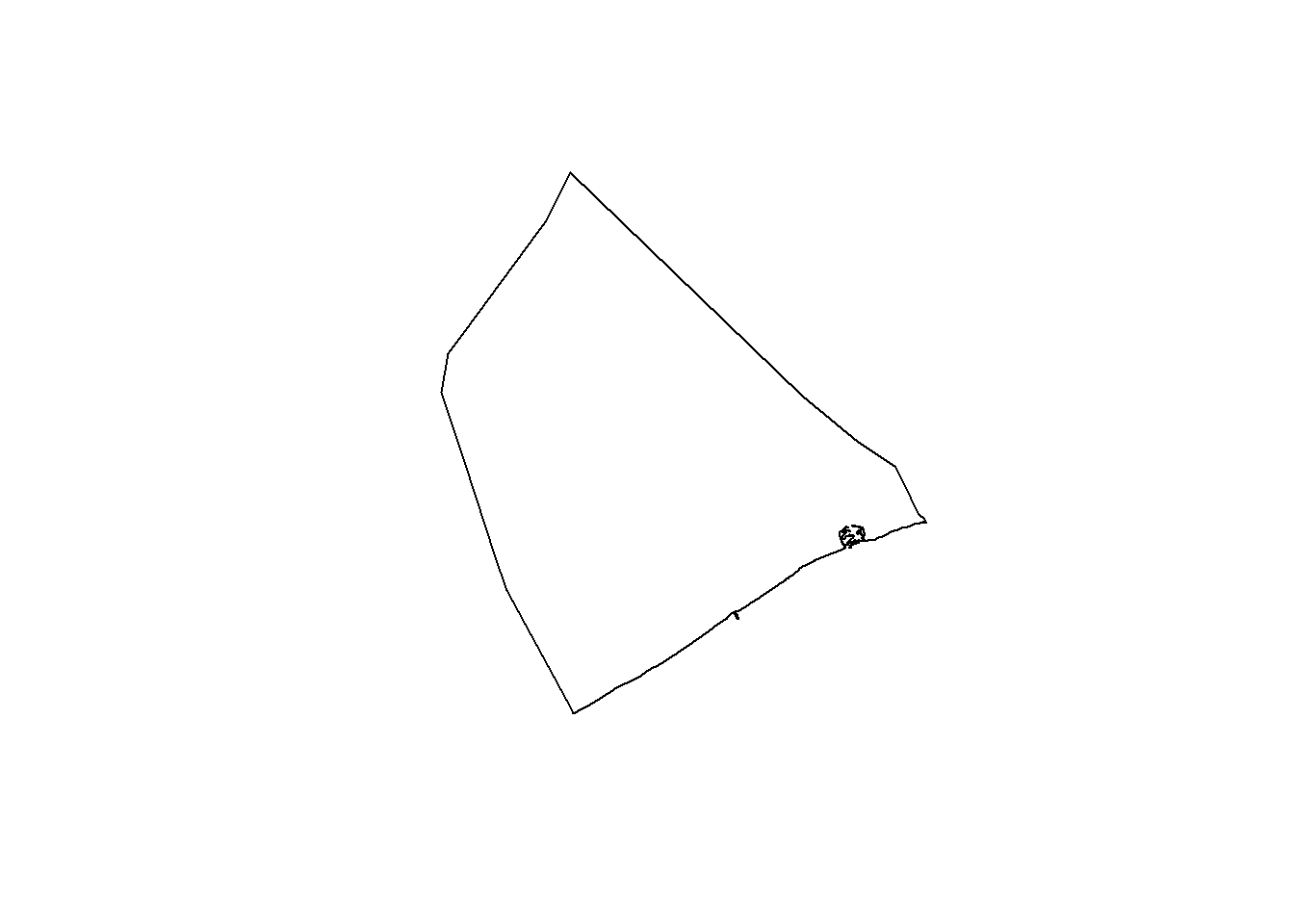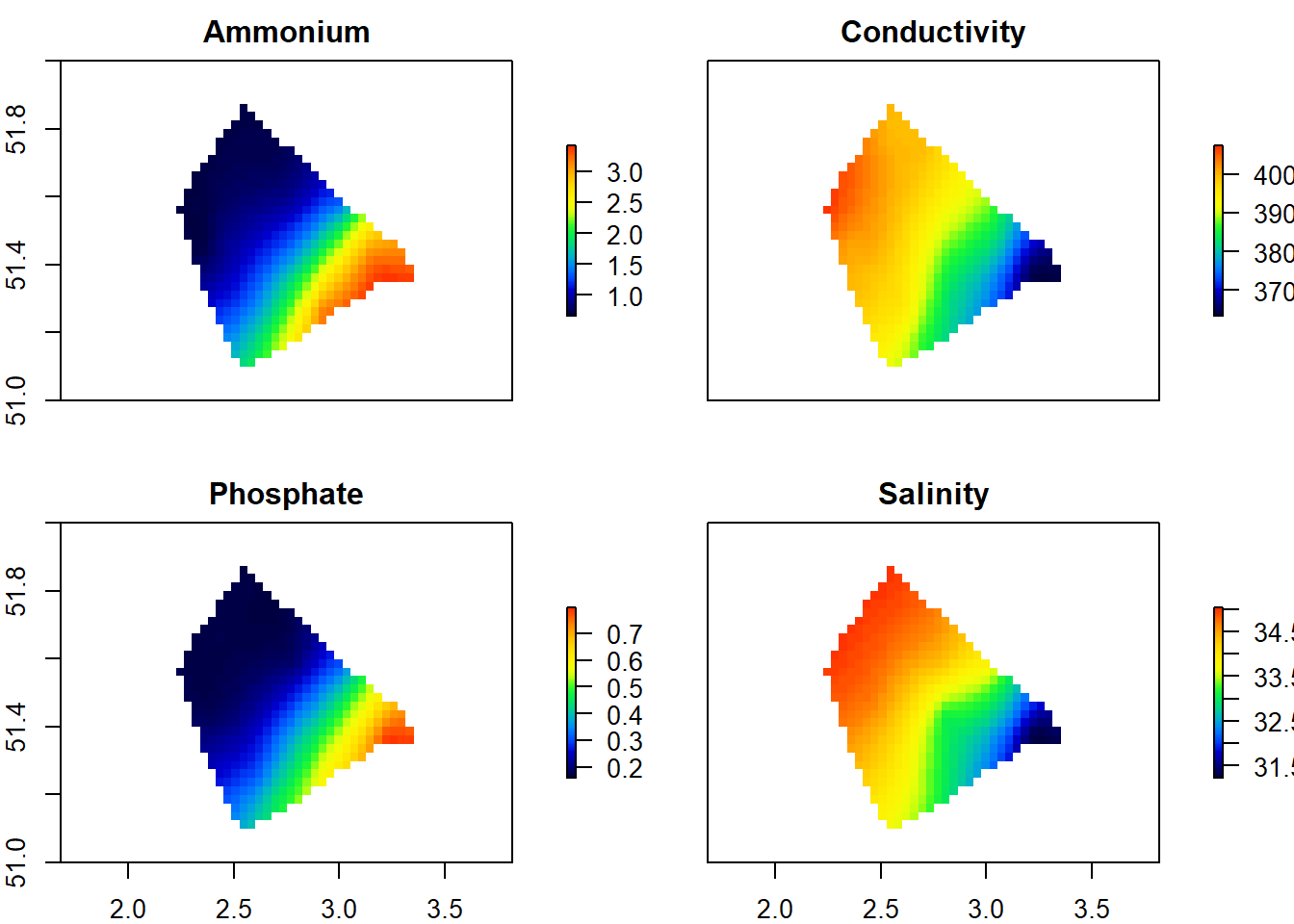Contributors: Written by Jonas Mortelmans (Flanders Marine Insitute) & Olivier Beauchard (University of Antwerp)
Source data: LifeWatch Station Data Explorer (https://rshiny.lifewatch.be/station-data/)
Institute: LifeWatch (www.lifewatch.be)

For various activities at sea, environmental data is needed. The 17 LifeWatch stations in the BPNS might be too far away from the location of interest to be of use, making them less representative for the specific location. Therefore, an interpolation exercise can be done for different parameters by using the station data. The output are interpolation maps covering the whole BPNS.

## 1. Install & load required packages

# install.packages("RColorBrewer", "raster", "mapdata", "maps", "gplots",
# "rgdal", "gstat", "prettymapr")
library(RColorBrewer)
library(raster)
library(mapdata)
library(maps)
library(gplots)
library(rgdal)
library(gstat)
library(prettymapr)
library(dplyr)
library(mregions)
library(rgdal)

## 2. Prepare data

data <- read.csv("./data/LW_data.csv", header=T, sep = ";")
# Read table in as tab file
View(data)

Group parameters per station, calculating the mean

data <- data %>%
group_by(Station) %>%
summarise_at(vars(-Time), funs(mean), na.rm = TRUE)

Delete the duplicate station w09, leaving us with 17 stations.

data <- filter(data, Station != "w09")

Create two datasets based on the data: one for the coordinates and one for the environmental parameters

coordinates <- data[,c(1:3)]
parameters <- data[,c(1,4:17)]

merged_data<- merge(coordinates,parameters, all = TRUE)

Change names of the columns

colnames(merged_data)[colnames(merged_data)=="Latitude"] <- "y"
colnames(merged_data)[colnames(merged_data)=="Longitude"] <- "x"

colnames(merged_data)[colnames(merged_data)=="Ammonium_NH4.Âµmol.N_NH4.L."] <- "Ammonium"
colnames(merged_data)[colnames(merged_data)=="Conductivity.mS.cm."] <- "Conductivity"
colnames(merged_data)[colnames(merged_data)=="Density.kg.m3."] <- "Density"
colnames(merged_data)[colnames(merged_data)=="Fluorescence.mg.mÂ.."] <- "Fluorescence"
colnames(merged_data)[colnames(merged_data)=="Nitrate_NO3.Âµmol.N_NO3.L."] <- "Nitrate"
colnames(merged_data)[colnames(merged_data)=="OBS.NTU."] <- "OBS"
colnames(merged_data)[colnames(merged_data)=="Pressure.db."] <- "Pressure"
colnames(merged_data)[colnames(merged_data)==
"Nitrate_Nitrite.Âµmol.N_NO3.NO2.L."] <- "Nitrate_Nitrite"
colnames(merged_data)[colnames(merged_data)=="Nitrite_NO2.Âµmol.N_NO2.L."] <- "Nitrite"
colnames(merged_data)[colnames(merged_data)=="Phosphate_PO4.Âµmol.P_PO4.L."] <- "Phosphate"
colnames(merged_data)[colnames(merged_data)=="Salinity.PSU."] <- "Salinity"

Delete ‘Station’ column

merged_data$Station <- NULL Subsets can be made from the dataset based on the preferred parameters for the interpolated maps. This is an example subsetting Ammonium concentration, Phosphate concentration & Salinity for the different stations (column 1:3, 13:14, 16) subset_data <- merged_data[,c(1:4,14,16)] Give the subset a new name (it’s also possible to use the full dataset) t <- subset_data t <- t[complete.cases(t),] ## 3. Build heatmaps First build your areal polygon: the region we are interested is the BPNS. Shapefile layers of the desired area can be downloaded from the Gazetteer on marineregions.org https://www.marineregions.org/gazetteer.php?p=search p <- readOGR("./eez_iho/eez_iho.shp", layer = "eez_iho") plot(p)Build 16 bricks and specify boundaries of your area of interest (here: BPNS) According to what subset you use, one can easily change the number of bricks, and the iteration (16 bricks-> iteration 3:18; 7 bricks -> iteration 3-9; etc). This will avoid having empty bricks. # We need to detach dplyr, a commonly used package which interferes with the code. detach("package:dplyr", unload=TRUE) b=brick(nl=4,nrows=40,ncols=40,xmn=2,xmx=3.5,ymn=51,ymx=52) for(i in 3:6){ # Columns can be adjusted based on how many interpolated maps you want to calculate. # here:Parameters from column 3:5 for the three parameters Ammonium, Phosphate & Salinity z=t[,i] coo=t[,c(2,1)] coo=coo[!is.na(z),] z=z[!is.na(z)] colnames(coo)=c("x","y") coordinates(coo)=~x+y # Grid topology: specify the size of your 'pixels'. # In this case we use pixels of 0.015. # If you change the pixels, you need to change the brick exent and number of pixels too # (calculate: 0.015 * 234 = 3.5 -> this is the height of one brick) g=GridTopology(c(2,51),c(0.008,0.008),c(187.5,125)) g=SpatialGrid(g) #feel free to modify these parameters until best heatmap is seen k=krige(z~1,coo,g,model=vgm(2000,"Sph",1000,0.1)) k=as(k,"SpatialPointsDataFrame") r=rasterize(k["var1.pred"],raster(nrows=40,ncols=40,xmn=2,xmx=3.5,ymn=51,ymx=52), fun=mean) r=mask(r$var1.pred,p)
b[[i-2]]=r\$var1.pred
names(b[[i-2]])=colnames(t)[[i]]
}
## [using ordinary kriging]
## [using ordinary kriging]
## [using ordinary kriging]
## [using ordinary kriging]

Edit maps by adding city - and river names

city=c("Nieuwpoort","Oostende","Zeebrugge")
xcity=c(2.75,2.92,3.24)
ycity=c(51.15,51.21,51.33)
rx=c(1.8,3.8)
ry=c(51.0,52.0)

river=c("Scheldt")
xriver=c(3.45)
yriver=c(51.48)

xstation=c(2.9047418,2.8500723,2.809427,2.7887353,2.6997728,
2.555862,2.4254687,2.2568947,2.3527137,2.449103,
2.5014,2.7016258,2.613685,3.0140097,3.0588778,3.138706,
3.2220728)
ystation=c(51.2712658,51.3088817,51.432511,51.5805838,51.7483265,
51.799364,51.6894492,51.5735387,51.4566392,51.4801112,
51.3326703,51.1853207,51.2768667,51.5885873,51.4707652,
51.4402072,51.3762588)
station=c(130,230,330,435,"W09","LW02","W10","LW01","W08",421,
"ZG02",120,215,"W07bis",780,710,700)

plot(b, col=rich.colors(100))Further edit the maps

fun <- function() {
#lty: line type (dash), #lwd: line width (breedte)
abline(h=seq(from=ry+0,to=ry-0,by=0.2),lty=1,lwd=0.1,col="gray")
abline(v=seq(from=rx+0,to=rx-0,by=0.2),lty=1,lwd=0.1,col="gray")

#add maps for Belgium outline, and the world

#scale

points(xcity,ycity,cex=1, pos=2)
text(xcity,ycity-0,city,pos=1,cex=2,font=3)

points(xstation,ystation,cex=1)

points(xriver,yriver,cex=0)
text(xriver-0.1,yriver+0.05,river,pos=1,cex=1.5,font=2, col="#366699",srt=-30)

}
jpeg("./figures/Test_Map.jpg",width=1500,height=1000)
dev.off()